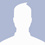# 7 Free A.I. Math Calculator Software With Step-by-Step Solver

Updated: June 11, 2019 /

Nobody loves math, unless it involves counting money with lots of zeros. Back in the good old days before computer and smartphones apps, the only way to solve an unsolvable mathematical problem is to ask your math teacher. You can solve calculus and algebra problems online with these free math calculators with steps to show your work.

Alternative 2020 Article ➤ 14 Funny Math Jokes and Meme Pictures

Here are some of the best Mathematics solving A.I. software for Advanced, Pure And Applied math. These applications and math programs not only solve your mathematical problems effortlessly but most important of all it shows the step by step method it took to reach the answer.

## ↓ 01 – SpeedCrunch | Windows | macOS | LinuxSpeedCrunch is a high-precision scientific calculator featuring a fast, keyboard-driven user interface. It is free and open-source software, licensed under the GPL. SpeedCrunch displays results as you type. Select a partial expression to evaluate only that part. Previous inputs and results are shown in a scrollable history and can be easily recalled. Customizable syntax highlighting allows you to spot mistakes easily.

SpeedCrunch comes with over 80 built-in mathematical functions. You can also define your own functions. Variables allow you to store your results. Perform calculations with up to 50 digits of precision, using complex numbers, numeral bases, unit conversions, and more.

• Unlimited Variables
• Syntax Highlighting
• Functions with ‘Automagic’ Completion
• 50 Decimal Precision

## ↓ 02 – SageMath | Web | Windows | macOS | LinuxSageMath is built out of nearly 100 open-source packages and features a unified interface. SageMath can be used to study elementary and advanced, pure and applied mathematics. This includes a huge range of mathematics, including basic algebra, calculus, elementary to very advanced number theory, cryptography, numerical computation, commutative algebra, group theory, combinatorics, graph theory, exact linear algebra and much more. It combines various software packages and seamlessly integrates their functionality into a common experience. It is well-suited for education and research.

Related Article :   4 Website Monetization Programs For Publishers With Moderate Web Traffic

## ↓ 03 – Photomath | Android | macOSPhotomath supports arithmetics, integers, fractions, decimal numbers, roots, algebraic expressions, linear equations/inequations, quadratic equations/inequations, absolute equations/inequations, systems of equations, logarithms, trigonometry, exponential and logarithmic functions, derivatives and integrals. Simply point your camera toward a math problem and Photomath will magically show the result with detailed step-by-step instructions. Children can use it as a tool which helps them to learn Math, while parents can use it to check the homework to their children. With PhotoMath, you can have a Math teacher in your pocket.

## ↓ 04 – Mathway | WebMathway provides students with the tools they need to understand and solve their math problems. With millions of users and billions of problems solved, Mathway is the #1 problem solving resource available for students, parents, and teachers. Mathway’s long-term goal is to make quality on-demand math assistance accessible to all students. Mathway is an ambitious, long-term creative endeavor that will deliver increasing features and functionality over the years to come. Mathway is like a private tutor in the palm of your hand, providing instant homework help anywhere, anytime. Mathway covers:

• Basic Math
• Pre-Algebra
• Algebra
• Trigonometry
• Precalculus
• Calculus
• Statistics
• Finite Math
• Linear Algebra
• Chemistry
• Graphing

## ↓ 05 – Cymath | WebEnter your math problem, and let Cymath solve it for you step-by-step! For additional help, you can join Cymath Plus for \$4.99 USD per month. With Cymath Plus, you can see how a step is done and why a step is taken on most problems and on a variety of topics. You can also enjoy Cymath ad-free. For high school students, Cymath covers topics in algebra such as:

• Equation solving
• Factoring
• Logarithms
• Exponents
• Complex numbers
• Trigonometry
• Partial fraction
• Polynomial division
Related Article :   8 Free Printable Educational Alphabet Flashcards For Kids

For college students, Cymath covers topics in calculus such as:

• Product rule
• Quotient rule
• Chain rule
• U-substitution
• Integration by parts
• Integration by partial fraction
• Trigonometric substitution
• Rationalizing substitution

## ↓ 06 – SymboLab Solver | WebSymbolab is an advanced math education tool. It allows users to learn, practice and discover math topics using mathematical symbols and scientific notations as well as text. Symbolab provides automated step by step solutions to algebraic, trigonometric and calculus topics covering from middle school through college. Symbolab offers a wealth of smart calculators including: equations, simultaneous equations, inequalities, integrals, derivatives, limits , tangent line, trigonometric equations, functions and more.

The stated goal of the site is to make scientific content universally accessible by expanding the searchable data space onto scientific notations, expressions, equations and formulas. This is done by applying proprietary machine learning algorithms in order to understand the meaning and context of the queries. Symbolab, making math simpler.

## ↓ 07 – Bing Search App | iOSWith the new ‘math mode’ in Bing’s Camera Intelligent Search feature in the iOS app, Bing can solve complex math problems for you with the snap of a picture. The coolest feature in this update is the new “Math mode” that uses Bing’s “Camera Intelligent search.” It allows anyone to easily solve complex math problems by simply taking a photo of the math problem. I tried it out, and it works pretty well, even on hard-to-read math problems and problems involving simpler addition and subtraction functions to problems involving algebra and calculus.

1.•